## Blog# Divide by Zero Errors

A common error that analysts experience is the divide by zero error

This article explains across a range of platforms how to avoid these issues with simple logic to make sure the division by zero errors do not get in the way of analysis

## Why can we not divide by zero?

Dividing by 0 makes no sense logically, this is because the result cannot be determined

Division by Zero | Brilliant Math & Science Wiki

So division by zero is something we must avoid

## What are the main ways to avoid dividing by zero?

If we focus on a few key widely used components of BI tools, the ways to avoid generally fall into these categories

• Check the denominator first, if it is zero then avoid the division and output a suitable value in its place
• Use a safe division type of option which has built in divide by zero handling

In most cases you can decide which option to use depending on what makes sense in the case you need it to apply to. Sometimes you will need to return a value of zero other times it may make more sense to show the value as NULL or even return a text message such as ‘N/A’

Lets look at common solutions for different software starting with data bases

## Divide by zero errors in SQL Server

Here we can see that if we divide by 0 in SQL Server we see the error

Divide by zero error encountered.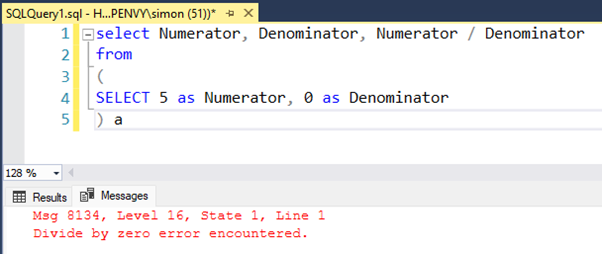### Use a case statement to fix Divide by zero in SQL Server

```Using a case statement can provide the most flexibility to handle different outputs in SQL server giving you the option to check for a zero value in the denominator first and output whatever you choose from a zero value to a NULL to a ‘Text Value’

We have added in an extra non zero denominated row for illustration

select Numerator, Denominator,
Case
When Denominator = 0 then 0
else Numerator / Denominator
end as Measure
from
(
SELECT 10 as Numerator, 0 as Denominator
union all
SELECT 10 as Numerator, 2 as Denominator
) a```

```To give this output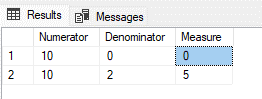```

Row 1 shows the measure as zero

alternatively if we chance the case statement logic to return a NULL

When Denominator = 0 then NULL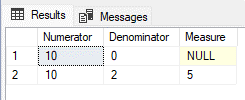### Use NULLIF to fix Divide by zero in SQL Server

```A simpler solution in SQL server if you are looking for a NULL output for divide by 0 is to use the NULLIF command around the denominator value

select Numerator, Denominator,
Numerator / NULLIF(Denominator,0) as Measure
from
(
SELECT 10 as Numerator, 0 as Denominator
union all
SELECT 10 as Numerator, 2 as Denominator
) a```

## Divide by zero in Google BigQuery

### Use a case statement to fix Divide by zero in Google BigQuery

The SQL for the case statement is exactly the same in Google BigQuery

for the zero output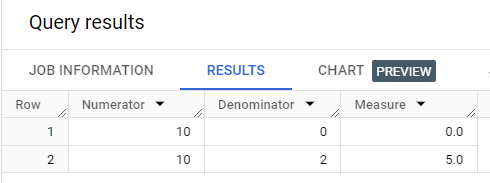For the NULL output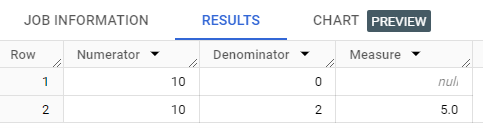### Use NULLIF to fix Divide by zero in Google BigQuery

The SQL for the case statement is exactly the same in Google BigQuery tooDepending on your query structure, there is a difference if you are checking for a NULL value

comparing SQL Server to BigQuery

In SQL Server      ISNULL(Denominator,0)

In BigQuery         IFNULL(Denominator,0)

## Divide by zero errors in Excel

If we continue with the same example in Excel

We have the formula in cell C2 as =A2/B2 then we see the #DIV/0! Error### using IFERROR in Excel

we can use the built-in IFERROR function to check for any errors in a formula and if there is an error we set the output value in its place

we change the formula to =IFERROR(A3/B3,0)

The value after the comma is the ‘value if error’ and determines what output value to use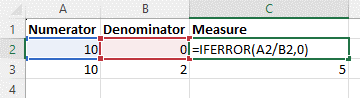Please note that IFERROR checks for all errors and if it finds any errors at all it then uses the substitute. With this in mind we don’t not regard this as a safe recommendation

### using IF statements in Excel

A better and safer option is to use IF statements in Excel, this way you can specifically check for a zero denominator

=IF(B3=0,0,A3/B3)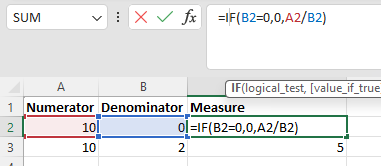This option can also give you the flexibility to use “” NULL values or text outputs

### Divide by zero in Power Pivot

Whilst still in Excel, hopping into Power Pivot

Assuming we have the same data as in Excel

We want to add a calculated field, we would recommend the IF statement option for the same reasons as in Excel, is that it is more specific

if([Denominator]=0,0,[Numerator]/[Denominator])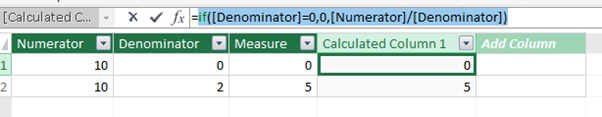You could ignore our advice and use the IFERROR function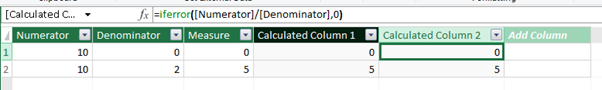But this would simply output a zero value for any error

## Divide by zero errors in Power BI

We get the divide by 0 in Power BI in both Power Query or DAX measures

To walk through our examples you can add the same data

In Power BI Desktop, click Enter Data

set the column headings to Numerator and Denominator and then enter the values as below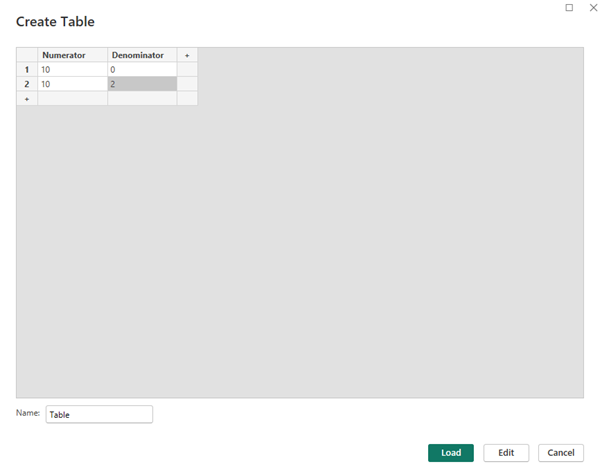### Divide by zero in Power Query

On the data pane, edit the query for this new table to open up Power Query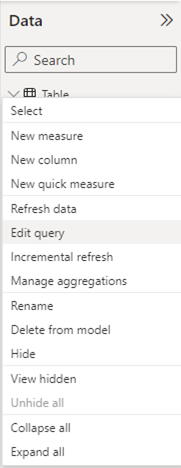You can see the table in Power Query

Use the conditional column feature to check if the divisor is zero and return a different value.

We will enter the [Numerator]/[Denominator] as a value to make it simpler to follow, then in the next step edit the M code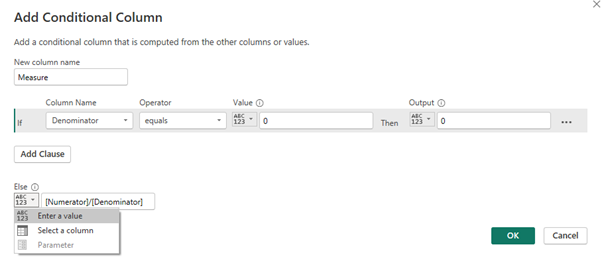Clicking OK nearly gives us what we are looking for, but the placeholder for the fraction needs to be updated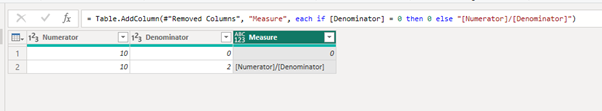The final step is to remove the quotes in the M Language code

= Table.AddColumn(#”Changed Type”, “Measure”, each if [Denominator] = 0 then 0 else [Numerator]/[Denominator])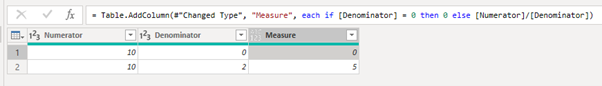### Divide by zero in DAX

We have a few different ways to solve the divide by 0 in DAX

Firstly create a simple table on the canvas with the Numerator and Denominator both set to Don’t Summarize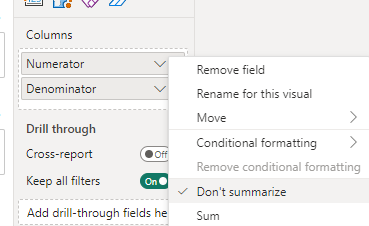It should look like this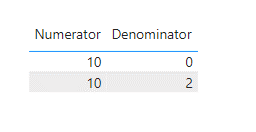We can now create the different measures

### Using DIVIDE in DAX

Create a new measure using this DAX code

Measure using DIVIDE = Divide(sum(‘Table'[Numerator]), sum(‘Table'[Denominator]), 0)

The DIVIDE function has a built-in safe division method, if the denominator is zero then it returns the final option, in our case above a zero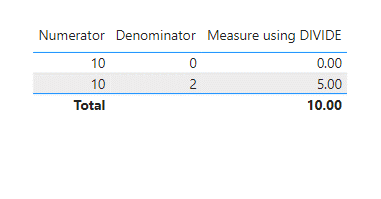### Using the IF function in DAX

Create a new measure using this DAX code

Measure using IF = if(sum(‘Table'[Denominator]) = 0, 0, sum(‘Table'[Numerator]) / sum(‘Table'[Denominator]))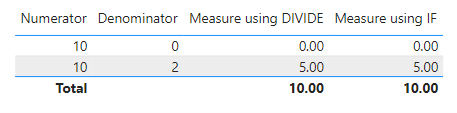## Conclusion

This is a small range of the use cases you may need to know to avoid the dreaded divide by zero errors, understanding these solutions is important to allow your analysis to be able to handle the divide by zero error

Subscribe to our channel to see more SQL tips and timesavers